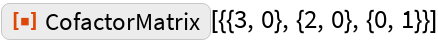#Function Repository Resource:

# CofactorMatrix

Give the matrix of cofactors for a given input matrix

Contributed by: Wolfram|Alpha Math Team
 ResourceFunction["CofactorMatrix"][mat] gives the cofactor matrix of the matrix mat.

## Examples

### Basic Examples

Find the matrix of cofactors of an input matrix:

 In:=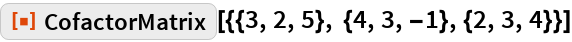Out=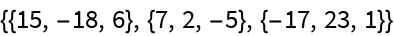The identity matrix is equal to its own cofactor matrix:

 In:=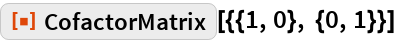Out=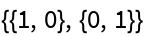Find the matrix of cofactors of another matrix:

 In:=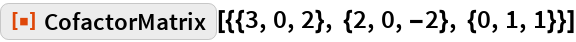Out=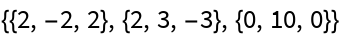Find the cofactor matrix of a matrix having symbolic elements:

 In:=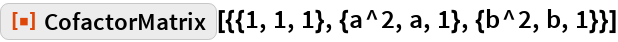Out=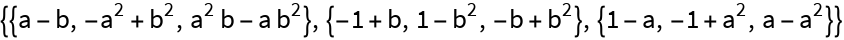### Scope

CofactorMatrix returns unevaluated for non-square matrix input:

 In:=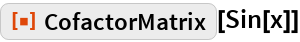Out=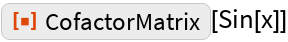In:=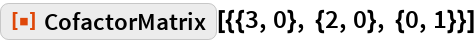Out=